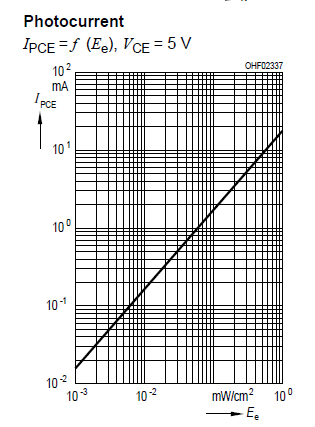# NPN Phototransistor SF313 and ADC

I accidentially got two SF313. They are phototransistors, here is a datasheet:

As far as I understand it if I apply +5V to it's collector-emmiter, the current running throw it will be proportional to the ammount of light (that's why it's have "High linearity" feature). Here is a drawing from datasheet:The dark current and photocurrent as far as I understand is max in the dark and max current with light respectively:

So my plan is to connect the following way:

+5V -> SFH Collector -> SFH Emmiter -> Some resistor to "convert" current to voltage -> (point of ADC connection) -> GND

Is this a right way to convert current changes to voltage changes? How to choose a resistor?

Is this a right way to convert current changes to voltage changes? How to choose a resistor?

Yes ! V = iR

Thanks for the reponse, should I choose a resistor based on max current (30 mA) in table above, row "Photocurrent"?

the current running throw it will be proportional to the ammount of light

Well that's not what the graph shows. It is a straight line graph alright but the axis are logarithmic. So the log of the current is proportional to the log of the light.

should I choose a resistor based on max current (30 mA)

Yes if you like.

Thanks Mike, yes, it seems graph is logarithmic. Maybe that's because of light nature?

May be there are any manuals how to use analog sensors with current change output with ADC?

Not manuals as such. But look around the web if you want to get more complicated than a single resistor. You can use op amps to linerise the output if you want.